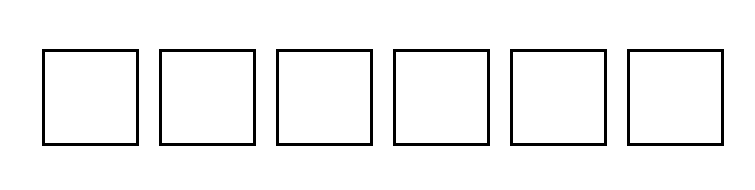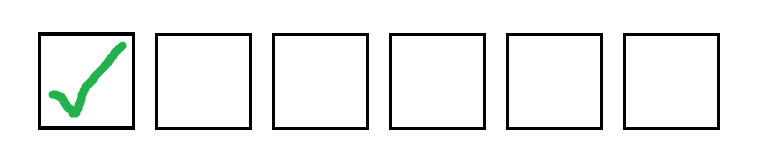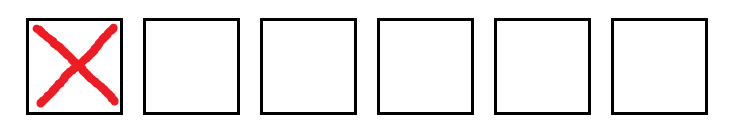# How does the casing method at around 2:00 work?

• Module 3 Week 2 Day 6 Challenge Part 4

How does the casing method at around 2:00 work? I still don't get it. What does that first box being x'ed or checked mean?

• @The-Blade-Dancer Good question! Basically, we're modelling the number "\(x\) choose \(y\)" or \(\binom{x}{y}\) as the number of ways to check off \(x\) boxes, if there are \(y\) boxes that can be checked.

Here, we have 6 boxes, and we want to find the number of ways to check 2 of them. Of course, you know that you can just do it using the formula for \(\binom{6}{2}\). But, we want to see if there is a different way to figure out this number, to find a different expression for this! That might lead to a new formula.

Here we are with the 6 boxes:You see the first box? We're either going to "check" it or not check it. There are the two possible "cases". If we count the number of ways in each of these two cases and add them together, we should get the total number of ways, \(\binom{6}{2}\).

What happens when we check it?In this case, if we know that we have to check the first box, how many ways are there to check off the rest? Well remember: We have to check 2 out of the 6 boxes. That means that we can only check off 1 more box. This means there are \(\binom{5}{1}\) ways to check off the rest, because we're really choosing 1 box to check out of the 5 boxes that remain. That's all for this case!

What happens if we don't check it?I'm putting a red X down to show that we are not allowed to check it in this case! But we still have 2 boxes left to check! And, there are 5 boxes remaining that we could check off. So, we need to choose 2 boxes to check from the 5 boxes remaining. That means there are \(\binom{5}{2}\) ways to check 2 boxes in this case.

We got \(\binom51\) ways in the first case and \(\binom52\) ways in the second case. These account for all the possibilities. That means that there are \(\binom51+\binom52\) ways in total to check off 2 boxes out of the 6. But we already knew that there were \(\binom62\) ways to do this! We count the same thing in two different ways. That leads us to this cool little formula:
\$\$\binom51+\binom52=\binom62\$\$
Of course, this trick doesn't just work for \(\binom62\). It works for anything! In fact, we have this general formula, called Pascal's Identity:
\$\$\binom{n-1}{k-1}+\binom{n-1}{k} = \binom{n}{k}\$\$
The example in the video showed this when \(n=6\) and \(k=2\). Try to prove that this is true for all numbers! All you have to do is take cases, like we did for finding a way to compute \(\binom{6}{2}\) in another way. It's just that instead of numbers, we're only using variables.

I hope that cleared things up!